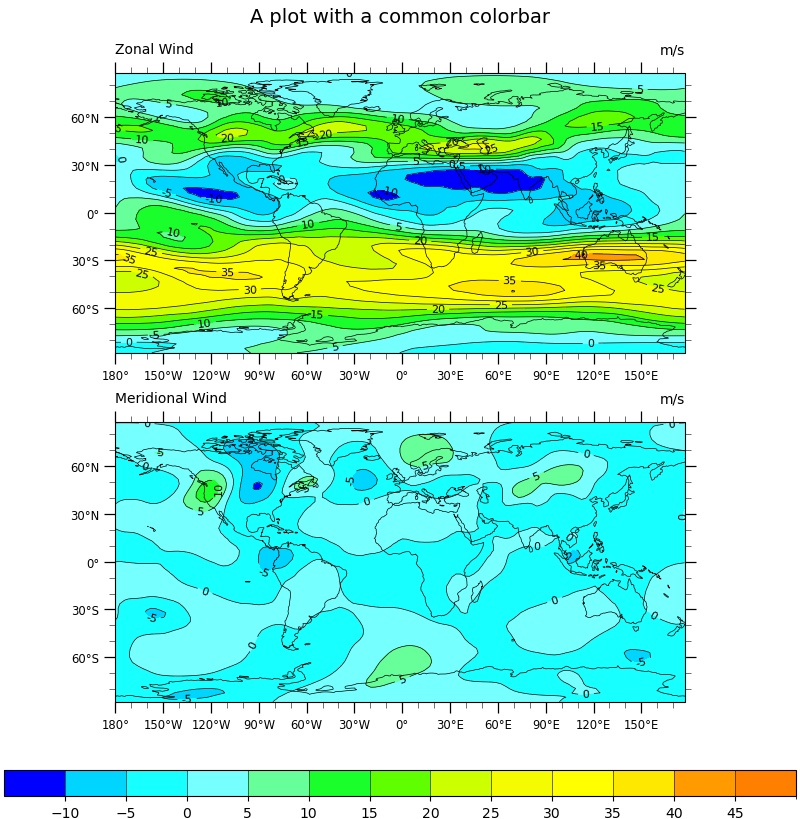# NCL_panel_3.py#

This script illustrates the following concepts:
• Two panel (subplot) image with shared colorbar and title

• Adding a common title to paneled plots

• Adding a common colorbar to paneled plots

• Importing and truncating a NCL colormap

See following URLs to see the reproduced NCL plot & script:

Import packages:

```import cartopy.crs as ccrs
import matplotlib.pyplot as plt
import numpy as np
import xarray as xr
import cmaps

import geocat.datafiles as gdf
import geocat.viz as gv
```

```# Open a netCDF data file using xarray default engine and load the data into xarrays, choosing the 2nd timestamp
ds = xr.open_dataset(gdf.get("netcdf_files/uv300.nc")).isel(time=1)
```

Utility Function: Labelled Filled Contour Plot:

```# Define a utility plotting function in order not to repeat many lines of codes since we need to make the same figure
# with two different variables.

def plot_labelled_filled_contours(data, ax=None):
"""A utility function for convenience that plots labelled, filled contours
with black contours marking each level.It will return a dictionary
containing three objects corresponding to the filled contours, the black
contours, and the contour labels."""

# Import an NCL colormap, truncating it by using geocat.viz.util convenience function
newcmp = gv.truncate_colormap(cmaps.gui_default, minval=0.03, maxval=0.8)

handles = dict()
handles["filled"] = data.plot.contourf(
ax=ax,  # this is the axes we want to plot to
cmap=newcmp,  # our special colormap
levels=levels,  # contour levels specified outside this function
xticks=np.arange(-180, 181, 30),  # nice x ticks
yticks=np.arange(-90, 91, 30),  # nice y ticks
transform=projection,  # data projection
add_labels=False,  # turn off xarray's automatic Lat, lon labels
)

# matplotlib's contourf doesn't let you specify the "edgecolors" (MATLAB terminology)
# instead we plot black contours on top of the filled contours
handles["contour"] = data.plot.contour(
ax=ax,
levels=levels,
colors="black",  # note plurals in this and following kwargs
linestyles="-",
linewidths=0.5,
add_labels=False,  # again turn off automatic labels
)

# Label the contours
ax.clabel(
handles["contour"],
fontsize=8,
fmt="%.0f",  # Turn off decimal points
)

ax.coastlines(linewidth=0.5)

# Use geocat.viz.util convenience function to add minor and major tick lines

# Use geocat.viz.util convenience function to make plots look like NCL plots by using latitude, longitude ticks

# Use geocat.viz.util convenience function to add main title as well as titles to left and right of the plot axes.
gv.set_titles_and_labels(ax,
lefttitle=data.attrs['long_name'],
lefttitlefontsize=10,
righttitle=data.attrs['units'],
righttitlefontsize=10)

return handles
```

Plot:

```# Make two panels (i.e. subplots in matplotlib)
# Specify ``constrained_layout=True`` to automatically layout panels, colorbars and axes decorations nicely.
# See https://matplotlib.org/tutorials/intermediate/constrainedlayout_guide.html
# Generate figure and axes using Cartopy projection
projection = ccrs.PlateCarree()
fig, ax = plt.subplots(2,
1,
constrained_layout=True,
subplot_kw={"projection": projection})

# Set figure size (width, height) in inches
fig.set_size_inches((8, 8.2))

# Define the contour levels
levels = np.linspace(-10, 50, 13)

# Contour-plot U data, save "handles" to add a colorbar later
handles = plot_labelled_filled_contours(ds.U, ax=ax)

# Set a common title
ax.set_title("A plot with a common colorbar", fontsize=14, y=1.15)

# Contour-plot V data
plot_labelled_filled_contours(ds.V, ax=ax)

cbar = plt.colorbar(
handles["filled"],
ax=ax,
orientation="horizontal",
ticks=levels[:-1],  # keep the last colorbar tick from showing
drawedges=True,
extendrect=True,
extendfrac='auto',
aspect=30)
cbar.ax.tick_params(labelsize=10)

# Show the plot
plt.show()
``````/home/docs/checkouts/readthedocs.org/user_builds/geocat-examples/conda/latest/lib/python3.10/site-packages/geocat/viz/util.py:692: UserWarning: Overwriting the cmap 'trunc(gui_default,0.03,0.80)' that was already in the registry.
mpl.colormaps.register(new_cmap, force=force)
```

Total running time of the script: ( 0 minutes 0.997 seconds)

Gallery generated by Sphinx-Gallery